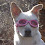## Friday, March 22, 2013

### Equations

For those of you who remember equations from your basic Algebra class, here's some homework to keep your brain firing on all cylinders:

1. Choose the best answer below for the following equation: Moonie + y = cat head in plate; where y is equal to a plate on the table with food item noted.

a. y = beef
b. y = fish
c. y = pork
d. y = all of the above

2. Choose the best answer below for the following equation:  x + Hugo = black cat pawing at food but never really touching it; where x is equal to something Hugo wants.
a. x = salmon
bx = pork
c. x = something, but he's not quite sure what it is, but he's sure that he wants it
d. x = all of the above

3Choose the best answer below for the following equation:   x + Ray = dog head in lap; where x is equal to a person eating the food item noted.

a.  x = peanut butter
b.  x = scones
c.  x = bacon
d. x = all of the above

4. Choose the best answer below for the following equation: x + y + z = the total annoyance level of person who's space is being violated. Where x, y, and z are annoyance level, on a ten point scale, caused by Moonie, Hugo, and Ray respectively for the above types of begging.
a. x = 8; y = 2; z = 5
b. x = 5; y = 5; z = 5
c. it doesn't really matter, we don't do anything about it anyway.
d. a and c
e. b and c

1. d
2. d
3. d
4. d

#### 1 comment:

1.Love it! I can especially relate to # 3 with the peanut butter.